## Sunday, September 12, 2021

### Transverse Waves: UNIZOR.COM - Physics4Teens - Waves

Notes to a video lecture on http://www.unizor.com

Transverse Wave

We are familiar with longitudinal waves, like sound waves in the air.
Their defining characteristic is that molecules of air (the medium) are oscillating along the direction of the wave propagation, which, in turn, causing oscillation of pressure at any point along the direction of wave propagation.

Consider a different type of waves.
Take a long rope by one end, stretching its length on the floor. Make a quick up and down movement of the rope's end that you hold.
The result will be a wave propagating along the rope, but the elements of rope will move up and down, perpendicularly to the direction of waves propagation.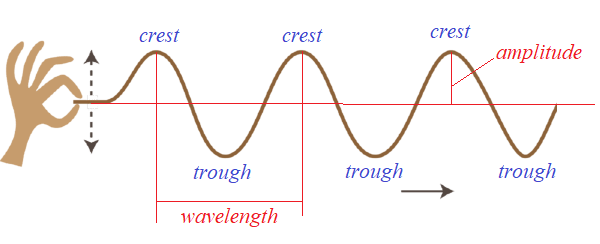(open this picture in a new tab of your browser by clicking the right button of a mouse to better see details)

These waves, when the elements of medium (a rope in our example) are moving perpendicularly to a direction of waves propagation, are called transverse.

Such elementary characteristics of transverse waves as crest, trough, wavelength and amplitude are clearly defined on the picture above.

Some other examples of transverse waves are strings of violin or any other string musical instrument.

Interesting waves are those on the surface of water. They seem to be transverse, but, actually, the movement of water molecules is more complex and constitutes an elliptical kind of motion in two directions - up and down perpendicularly to waves propagation and back and forth along this direction.

Our first problem in analyzing transverse waves is to come up with a model that resembles the real thing (like waves on a rope), but yielding to some analytical approach.

Let's model a rope as a set of very small elements that have certain mass and connected by very short weightless links - sort of a long necklace of beads.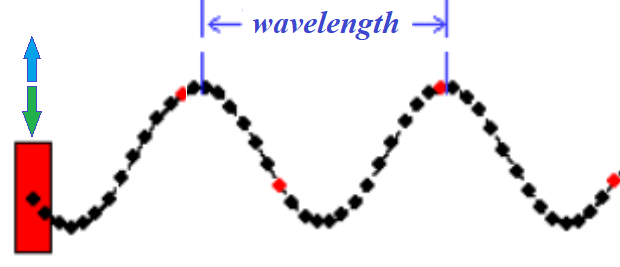Every bead on this necklace is a point-object of mass m, every link between beads is a solid weightless rod of length r.
Both mass of an individual bead m and length of each link r are, presumably, very small. In theory, it would be appropriate to assume them to be infinitesimally small.

Even this model is too complex to analyze. Let's start with a simpler case of only two beads linked by a solid weightless rod.
Our purpose is not to present a complete analytical picture of waves, using this model, but to demonstrate that waves exist and that they propagate.

Consider the following details of this model.
Two identical point-objects α and β of mass m each on the coordinate plane with no friction are connected with a solid weightless rod of length r.

Let's assume that α, initially, is at coordinates (0,−A), where A is some positive number and β is at coordinates (a, −A).
So, both are at level y=−A, separated by a horizontal rod of length r from x=0 for α to x=a for β.

We will analyze what happens if we move the point-object α up and down along the vertical Y-axis (perpendicular to X-axis), according to some periodic oscillations, like
yα(t) = −A·cos(ω·t)
where
A is an amplitude of oscillations,
ω is angular frequency,
t is time.
Incidentally, for an angular frequency ω the period of oscillation is T=2π/ω.
So, the oscillations of point α can be described as
yα(t) = −A·cos(2π·t / T)

Point α in this model moves along the Y-axis between y=−A and y=A.
It's speed is
y'α(t) = A·ω·sin(ω·t) =
= A·(2π/T)·sin(2π·t/T)

The period of oscillations is, as we noted above
T = 2π/ω

During the first quarter of a period α moves up, increasing its speed from v=0 at y=−A to v=A·ω at the point y=0, then during the next quarter of a period it continues going up, but its speed will decrease from v=A·ω to v=0 at the top most point y=A.
During the third quarter of a period α moves down from y=A, increasing absolute value of its speed in the negative direction of the Y-axis from v=0 to the same v=A·ω at y=0, then during the fourth quarter of a period it continues going down, but the absolute value of its speed will decrease from v=A·ω to v=0 at the bottom y=−A.

Positions of objects α and β during the first quarter of a period of motion of α at three consecutive moments in time are presented below.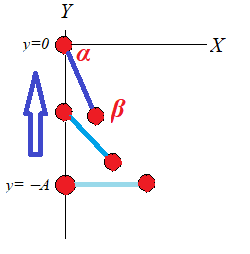As the leading object α starts moving up along Y-axis, the led by it object β follows it, as seen on a picture above.

Let's analyze the forces acting on each object in this model.
Object β is moved by two forces: tension from the solid rod Tβ(t), directed along the rod towards variable position of α, and constant weight Pβ.
Object α experiences the tension force Tα(t), which is exact opposite to Tβ(t), the constant weight Pα and pulling force Fα(t) that moves an entire system up and down.

There is a very important detail that can be inferred from analyzing these forces.
The tension force Tα(t), acting on object α, has vertical and horizontal components from which follows that pulling force Fα(t) cannot be strictly vertical to move object α along the Y-axis, it must have a horizontal component to neutralize the horizontal component of Tα(t).

This can be achieved by having some railing along the Y-axis that prevents α to deviate from the vertical path. The reaction of railing will always neutralize the horizontal component of the Tα(t). Without this railing the pulling force Fα(t) must have a horizontal component to keep α on the vertical path along the Y-axis.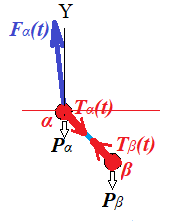If α and β are the first and the second beads on a necklace, we can arrange the railing for α. But, if we continue our model and analyze the movement of the third bead γ attached to β, there can be no railing and the horizontal component of the tension force Tβ(t) will exist and will get involved on some small scale.

All-in-all, transverse motion is not just movement of components up and down perpendicularly to the wave propagation, it is also a longitudinal motion of these components, though not very significant in comparison with transverse motion and often ignored in textbooks.

The really obvious reason for transverse motion to involve a minor horizontal movement in addition to a major vertical one is that you cannot lift up a part of a horizontally stretched rope without a little horizontal shift of its parts as well, because a straight line is always shorter than a curve.

Let's now follow the motion of β as α periodically moves up and down, starting at point (0,−A), according to a formula
yα(t) = −A·cos(ω·t)
with its X-coordinate always being equal to zero.

Since position of α is specified as a function of time and the length of a rod connecting α and β is fixed and equal to r, position of β can be expressed in term of a single variable - the angle φ(t) from a vector parallel to a rod directed from α to β and the positive direction of the Y-axis.
At initial position, when the rod is horizontal, φ(0)=π/2.

Then coordinates of β are:
xβ(t) = r·sin(φ(t))
yβ(t) = yα(t) + r·cos(φ(t))

During the first quarter of a period α moves up from y=−A to y=0, gradually increasing its speed and pulling β by the rod upwards.

While β follows α up, it also moves closer to the Y-axis. The reason for this is that the only forces acting on β are the tension force along the rod and its weight.
Weight is vertical force, while tension acts along the rod and it has vertical (up) and horizontal (left) components. Assuming vertical pull is sufficient to overcome the weight, β will be pulled up and to the left, closer to the Y-axis.

If this process of constant acceleration of α continued indefinitely, β would be pulled up and asymptotically close to the Y-axis. Eventually, it will just follow α along almost the same vertical trajectory upward.
The angle φ(t) in this process would gradually approach π (180°).

With a periodic movement of α up and down the Y-axis, the trajectory of β is much more complex.
Let's analyze the second quarter of the period of α's oscillations, when it moves from y=0 level, crossing the X-axis, to y=A.

After α crosses the X-axis it starts to slow down, decelerate, while still moving up the Y-axis.
As α decelerates in its vertical motion up, the composition of forces changes.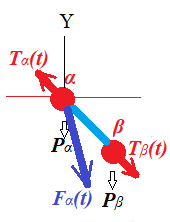Now β will continue following α upwards, but, instead of being pulled by the rod, it will push the rod since α slows down.

That will result in the force Tα(t), with which a rod pushes α, to be directed upwards and a little to the left, as presented on the above picture.
Also Tβ(t), the reaction of the rod onto β, will act opposite to a tension during the first quarter of a period. Now it has a vertical component down to decelerate β's upward motion and a horizontal component to the right.

The latter will cause β to start moving away from the Y-axis, while still going up for some time, slowing this upward movement because of opposite force of reaction of the rod.

When α reaches level y=A at the end of the second quarter of its period, it momentarily stops.
The behavior of β at that time depends on many factors - its mass m, amplitude A and angular frequency ω of α's oscillations, the length of the rod r.
Fast moving leading object α will usually result in a longer trajectory for β, while slow α will cause β to stop sooner.

During the third quarter of its period α starts moving downward, which will cause β to follow it, but not immediately because of inertia. Depending on factors described above, β might go up even higher than α. In any case, the delay in β's reaching its maximum after α has completed the second quarter of a period will exist, that would cause β to reach its maximum later than α.

The delay between reaching its maximum height by β is the source of a visual effect that call waves. Every subsequent bead on a necklace will reach its top height a little later than the previous.

Assuming that α accelerates faster than g on its way down during the third quarter of a period, α will pull β down and closer to the Y-axis. Situation will be similar to the first quarter of a period, when α accelerates up.
Then during the fourth quarter α will slow down to full stop, while inertia and weight move β faster down, so it will have to make a small circle around the last level of α before a new period starts, and α pulls β again up.

The above relatively complicated description of the trajectory of β is supposed to serve as an explanation of the fact that this trajectory represents not simply an up and down motion of beads of a necklace in our model of the transverse oscillations, but is much more complex motion that involves both transverse and longitudinal oscillations with former playing a major role, while latter, however small, is still an important part of a motion.